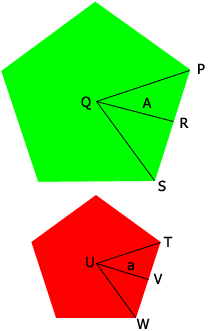SEARCH HOMEMath Central Quandaries & QueriesQuestion from Kip: The ratio of the corresponding apothems of two similar regular pentagons is 5:7 What is the ratio of their areas?Hi Kip,

In my diagram the apothems are of length $A$ and $a$ where $A:a = 7:5.$ Notice also that triangles $PQR$ and $TUV$ are similar.Now look at our response to a question from Jenna.

Penny* Registered trade mark of Imperial Oil Limited. Used under license.Math Central is supported by the University of Regina and the Imperial Oil Foundation.